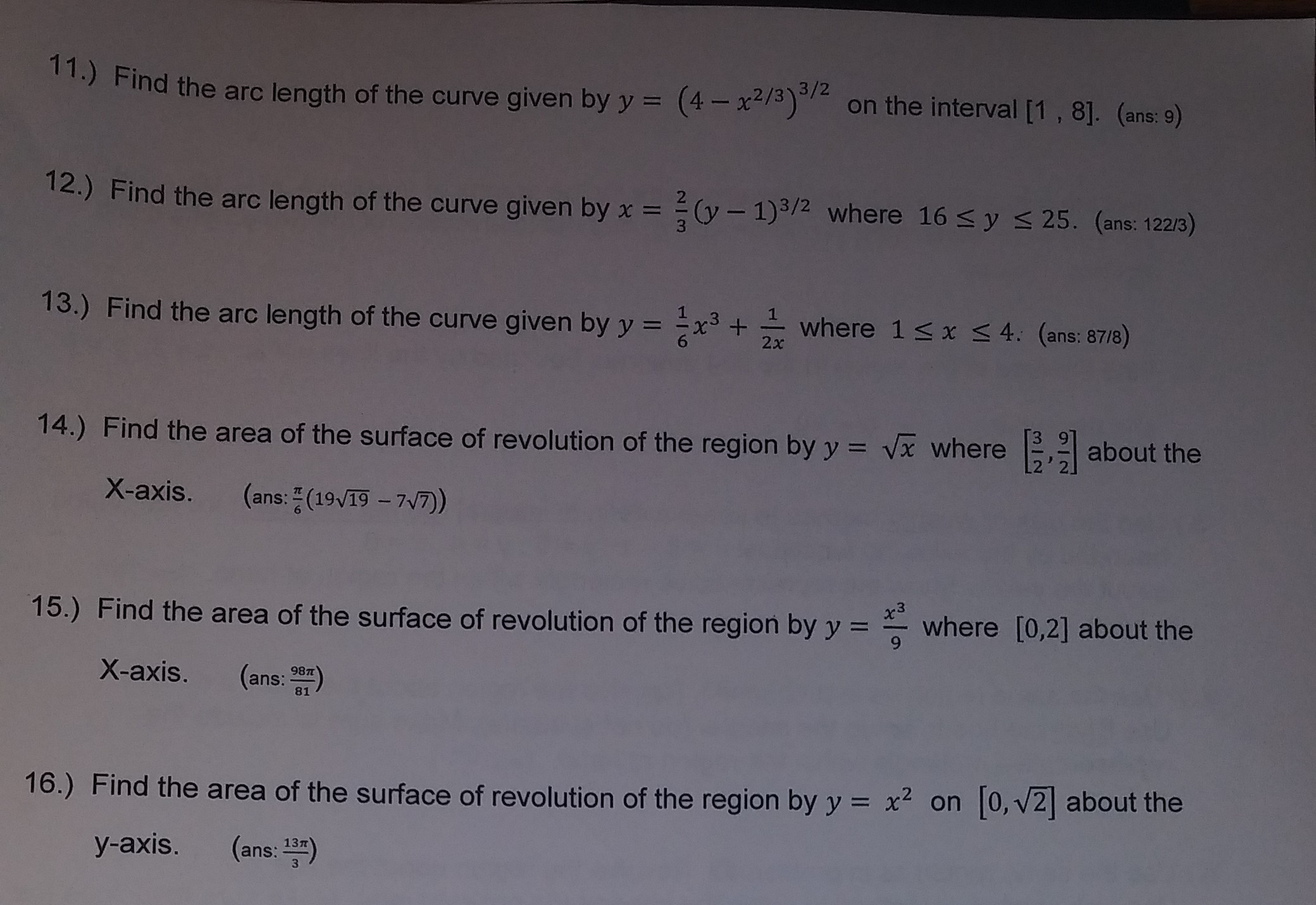11.) Find the arc length of the curve given by y (4on the interval [1, 81. (ome:-)3/2(4x2/3oon the interval [1, 8]. (ans: 9)Find the arc length of the curve given by x-2 (y - 1)3/2 where 16 s y s 25. (ans: 122/3)13.) Find the arc length of the curve given byyx where 1 s x 4. (ans: 8718)14.) Find the area of the surface of revolution of the region by yvx whereabout theX-axis. (ans: (1917)3where [0,2] about theFind the area of the surface of revolution of the region by yX-axis. (ans: a)15.)16,) Find the area of the surface of revolution of the region by y 2 on [o,v2] about they-axis. (ans: 13)

Question

Number 16help_outlineImage Transcriptionclose11.) Find the arc length of the curve given by y (4on the interval [1, 81. (ome:-) 3/2 (4x2/3o on the interval [1, 8]. (ans: 9) Find the arc length of the curve given by x- 2 (y - 1)3/2 where 16 s y s 25. (ans: 122/3) 13.) Find the arc length of the curve given byyx where 1 s x 4. (ans: 8718) 14.) Find the area of the surface of revolution of the region by y vx where about the X-axis. (ans: (1917) 3 where [0,2] about the Find the area of the surface of revolution of the region by y X-axis. (ans: a) 15.) 16,) Find the area of the surface of revolution of the region by y 2 on [o,v2] about the y-axis. (ans: 13) fullscreen
Step 1

If a curve y = f(x), where a ≤ x ≤ b is revolved about y axis then the area of the resulting surface is given by the below mentioned formula.

Here given curve equation y = x2 and 0 ≤ x ≤ √2.

Step 2

y = x2. So differentiating it with respect to x we get the following

Now substituting the ...

Want to see the full answer?

See Solution

Want to see this answer and more?

Our solutions are written by experts, many with advanced degrees, and available 24/7

See Solution
Tagged in

Calculus